A plastic box of mass 5 kg is found to accelerate up at the rate of g / 6, when placed deep inside the water. How much sand should be put inside the box so that it may accelerate down at the rate of g / 6?

1.  2kg

2.  3kg

3.  4kg

4.  5kg

Concept Questions :-

Application of laws
High Yielding Test Series + Question Bank - NEET 2020

Difficulty Level:

The graph for stopping time (t) versus mass (m) is shown in the figure. Then which one of the pair is given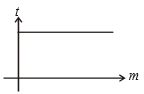1.  Momentum, force

2.  K.E., force

3.  Velocity, force

4.  None of these

Concept Questions :-

Application of laws
High Yielding Test Series + Question Bank - NEET 2020

Difficulty Level:

Tension on the string at point P is T. The graph for tension (T) versus x is shown in the figure. Then the string is: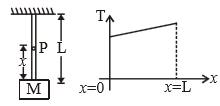1.  massless

2.  massfull

3.  tension on every point on the string is same when the string is having finite mass 4

4.  None of these

Concept Questions :-

Application of laws
High Yielding Test Series + Question Bank - NEET 2020

Difficulty Level:

A block is kept on a rough inclined plane with angle of inclination $\theta$. The graph of net reaction (R) versus $\theta$ is:

1.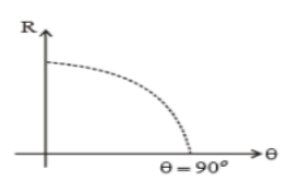2.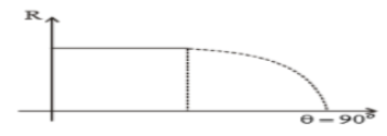3.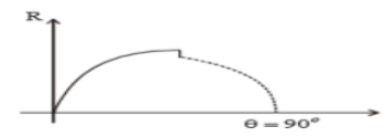(4) None of these

Concept Questions :-

Application of laws
High Yielding Test Series + Question Bank - NEET 2020

Difficulty Level:

A block is placed on a rough horizontal plane. A time dependent horizontal force F=kt acts on the block. The acceleration time graph of the block is :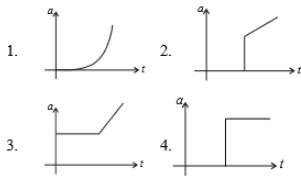Concept Questions :-

Application of laws
High Yielding Test Series + Question Bank - NEET 2020

Difficulty Level:

A rod of length L and mass m is acted on by two unequal forces ${F}_{1}$ and ${F}_{2}\left(<{F}_{1}\right)$ as shown in the following figure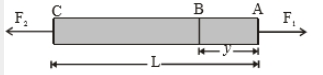The tension in the rod at a distance y from the end A is given by :

1. ${F}_{1}\left(1-\frac{y}{L}\right)+{F}_{2}\left(\frac{y}{L}\right)$

2. ${F}_{2}\left(1-\frac{y}{L}\right)+{F}_{1}\left(\frac{y}{L}\right)$

3. $\left({F}_{1}-{F}_{2}\right)\frac{y}{L}$

4. None of the above

Concept Questions :-

Application of laws
High Yielding Test Series + Question Bank - NEET 2020

Difficulty Level:

Two blocks A and B of masses m & 2m respectively are held at rest such that the spring is in natural length. Find the accelerations of both the blocks just after release.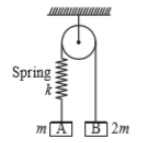(1)

(2)

(3) (0, 0)

(4)

Concept Questions :-

Application of laws
High Yielding Test Series + Question Bank - NEET 2020

Difficulty Level:

Two blocks 'A' and 'B' each of mass 'm' are placed on a smooth horizontal surface. Two horizontal force F and 2F are applied on both the blocks 'A' and 'B' respectively as shown in figure. The block A does not slide on block B. Then the normal reaction acting between the two blocks is: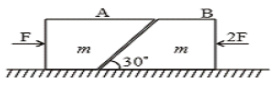(1) F

(2) F/2

(3) $\frac{F}{\sqrt{3}}$

(4) 3F

Concept Questions :-

Application of laws
High Yielding Test Series + Question Bank - NEET 2020

Difficulty Level:

Five persons A, B, C, D & E are pulling a cart of mass 100 kg on a smooth surface and the cart is moving with acceleration 3 $\mathrm{m}/{\mathrm{s}}^{2}$ in east direction. When person 'A' stops pulling, it moves with acceleration 1 $\mathrm{m}/{\mathrm{s}}^{2}$ in the west direction. When person 'B' stops pulling, it moves with acceleration 24 $\mathrm{m}/{\mathrm{s}}^{2}$ in the north direction. The magnitude of the acceleration of the cart when only A & B pull the cart keeping their directions same as the old directions are:

1.  26 $\mathrm{m}/{\mathrm{s}}^{2}$

2.

3.  25 $\mathrm{m}/{\mathrm{s}}^{2}$

4.  30 $\mathrm{m}/{\mathrm{s}}^{2}$

Concept Questions :-

Application of laws
High Yielding Test Series + Question Bank - NEET 2020

Difficulty Level:

Two masses m and M are connected by a light string passing over a smooth pulley. When mass m moves up by 1.4 m in 2 sec, the ratio $\frac{m}{M}$ is

(1) $\frac{13}{15}$

(2) $\frac{15}{13}$

(3) $\frac{9}{7}$

(4) $\frac{7}{9}$

Concept Questions :-

Application of laws